Function Repository Resource:

# PosetQ

Determine if a set of coordinates is partially ordered

Contributed by: Ed Pegg Jr
 ResourceFunction["PosetQ"][poset] determines if the coordinates in poset are partially ordered.

## Details

A poset is a partially ordered set of integer coordinates.

## Examples

### Basic Examples (3)

Test various posets:

 In:=In:=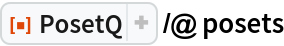Out=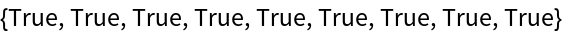Test various non-posets:

 In:=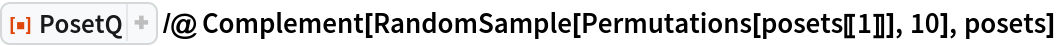Out=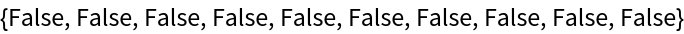For PosetQ, posets must be comprised of integers:

 In:=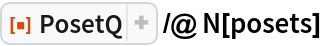Out=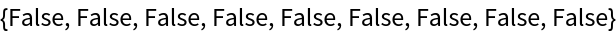### Scope (4)

For PosetQ, posets represent locations of values in a Young tableau:

 In:=Out=Here are the corresponding posets:

 In:=Out=Happily, they are identified as posets:

 In:=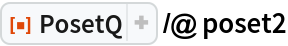Out=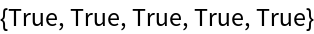Discounting n! problems, PosetQ can be used to find posets:

 In:=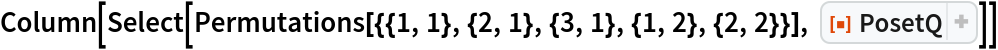Out=### Possible Issues (2)

This function does not work with the posets used in HasseDiagram:

 In:=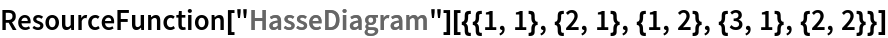Out=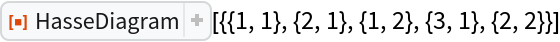This is a valid partial ordering, but it is not coordinate based:

 In:=Out=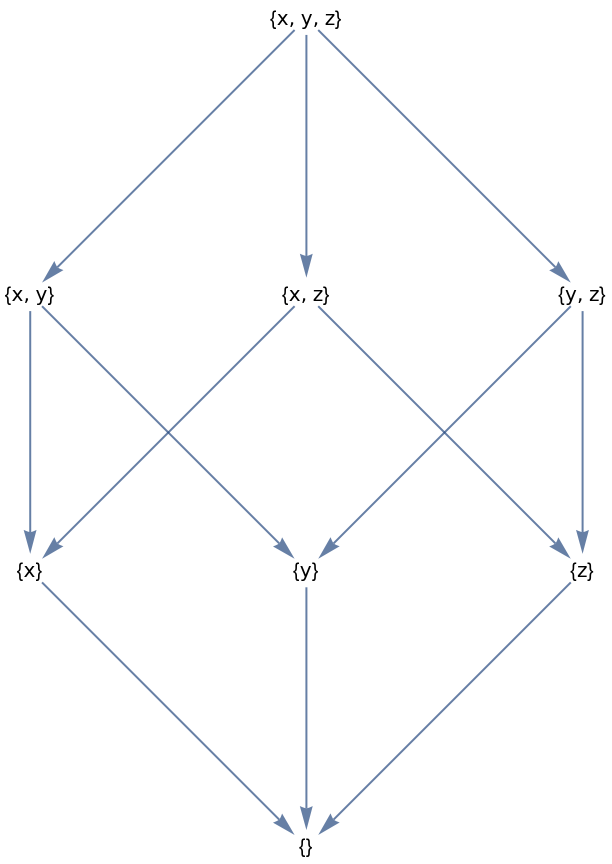## Version History

• 1.0.0 – 28 February 2022